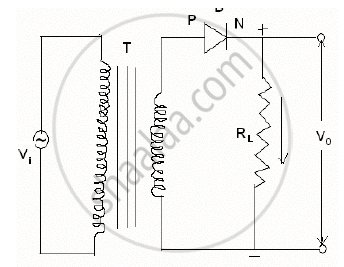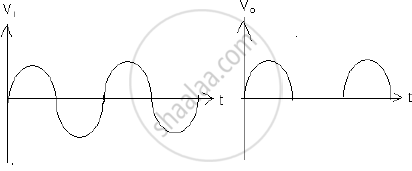Share

# With the Help of Neat Labelled Circuit Diagram Explain The Working of Half Wave Rectifier Using Semiconductor Diode. - Physics

#### Question

With the help of neat labelled circuit diagram explain the working of half wave rectifier using semiconductor diode. Draw the input and output waveforms.

#### Solution

A device which converts A.C. to D.C. is called rectifier. In this case output exists only for half cycle hence it is called half wave rectifier. Construction: The circuit diagram of a half wave rectifier using a junctiondiode is as shown in fig. The alternating voltage source is connected to the primary coil of a transformer. The secondary coil is connected to the diode in series with a resistance RL called the load resistanceT=Transformer

D=Diode

V0=output voltage

v1=input votage

Working: In first cycle of input voltage, the anode of the diode is positive potential w.r.t. cathode. Hence the diode is in forward-biased. Hence it conduct current. The current flows through load resistance giving voltage drop iRL. This voltage drop is called output voltage. During next half cycle the anode of diode is in negative potential w.r.t. Hence it is in reversed-biased. Hence it does not conduct the current. Hence current does not flow through load resistance giving no P.D. across it. Hence output voltage is unidirectional. It is called as D.C.

Is there an error in this question or solution?

#### APPEARS IN

2012-2013 (March) (with solutions)
Question 7.1 | 3.00 marks
2016-2017 (March) (with solutions)
Question 7.1 | 3.00 marks

#### Video TutorialsVIEW ALL 

Solution With the Help of Neat Labelled Circuit Diagram Explain The Working of Half Wave Rectifier Using Semiconductor Diode. Concept: Semiconductor Diode.
S